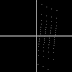In this article you will learn about tan function. How to draw tan function on an image with PHP code. Mostly these functions are used in mathematics, computer pixels and Numerical Computation. Watch complete code with example below and run on any server to watch results this tangent function.

```<?php
\$x = 600;
\$y = 315;
\$gd = imagecreatetruecolor(\$x, \$y);   //create background image
\$red = imagecolorallocate(\$gd, 255, 255, 255);   //create image
for (\$i = 0; \$i < 600; \$i++) {
imagesetpixel(\$gd, 300, \$i, \$red);  //draw vertical line
imagesetpixel(\$gd, \$i, 157, \$red);  //draw horizontal line
}
\$x = 0;
for(\$i = 90; \$i <= 360; \$i+=3)
{
\$y =  157 + (50*tan(\$i));   //get y-axis with farmula
imagesetpixel(\$gd, \$x+300, \$y, \$red);  //draw elips
\$x++;
}
PHP code to draw tan function on an imageReviewed by Unknown on 01:49 Rating: 5